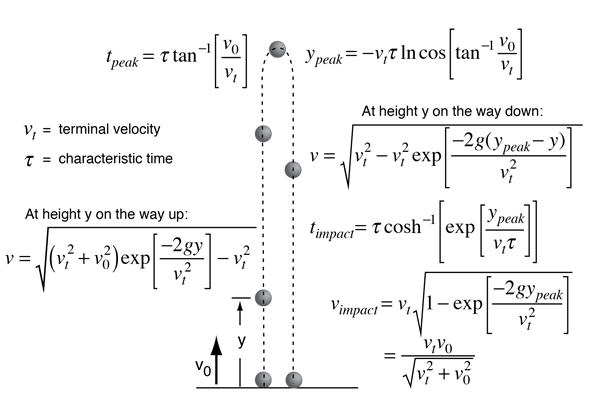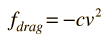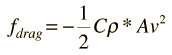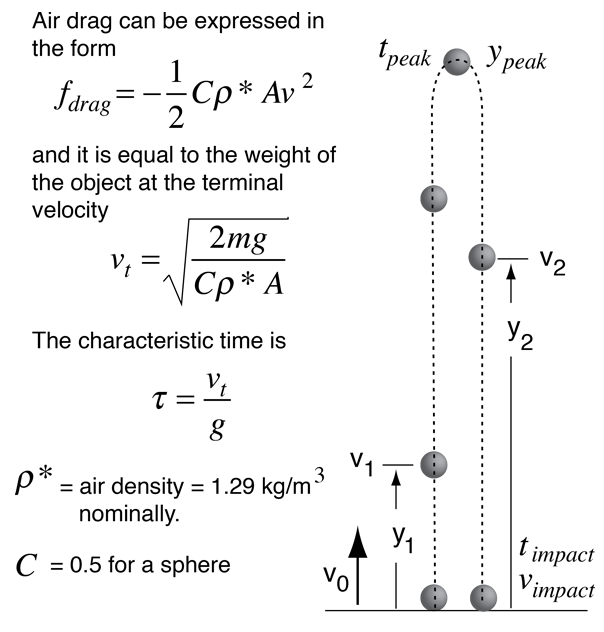# Vertical Trajectory

Objects moving at high speeds through air encounter air drag proportional to the square of the velocity. Describing the motion of objects under this quadratic drag usually requires numerical techniques rather than straight analytic formuli since the drag force and the gravitational force are not acting along the same line. The case of the vertical trajectory can be treated analytically since the forces are colinear. It is common practice to express the velocity and time in terms of the terminal velocity vt and a characteristic time τ. The characteristic time is defined as the terminal velocity divided by the acceleration of gravity, τ = vt/g.

Click on a formula for its developmentTwo common approaches to the quadratic drag force are:

1. Express the drag in terms of a single drag coefficient c:Calculation
2. Assume that drag is proportional to cross-sectional area and express in terms of area and a shape-dependent drag coefficient C:Calculation
Index

Fluid friction

 HyperPhysics***** Mechanics ***** Fluids R Nave
Go Back

# Vertical Trajectory Calculation

This is a model calculation for a vertical trajectory of a sphere of radius
r = cm and density ρ = kg/m3 = gm/cm3

the mass is m = gm.Assume the object has a drag coefficient C = (the default value is C = 0.5 for a sphere) and is falling through air with density ρ* = kg/m3 (the default value is 1.29 kg/m3).

Under these conditions, it's terminal velocity will be
vt = m/s
vt = km/hr
vt = mi/hr

and its characteristic time is
τ = s.

If this sphere is launched vertically with a velocity of
v0 = m/s = ft/s

then on the way up at height y1 = m = ft
it will have velocity v1 = m/s = ft/s

It will reach a peak height ypeak = m = ft
at time tpeak = s

On the way down at height y2 = m = ft
it will have velocity v2 = m/s = ft/s

It will reach the ground at velocity
vimpact = m/s = ft/s = km/hr = mi/hr
at time timpact = s after its peak height.

For comparison, if there were no air friction the projectile would have reached height
h = m = ft
and would impact with the original launch velocity at time t = s.

Index

Fluid friction

 HyperPhysics***** Mechanics ***** Fluids R Nave
Go Back